# Cryptography and Number Theory

## The Art of the Hidden Message: The role of number theory and prime numbers in online security

Online security presents new challenges for security. Let’s see how some discoveries in mathematics unexpectedly made the age of secure online transactions possible.  Some of the mathematics in the section on prime numbers and Fermat’s Little Theorem, and the encryption scheme known as RSA, are on the level of a typical intermediate level university mathematics course; if you have a few hours and want to understand exactly how this encryption scheme works, you can work through the mathematics of this.   Otherwise, feel free to read through the beginnings of these sections, and skim through the more technical parts and head to the final section for a brief outline of the mathematics involved.

### Introduction: how ‘pure’ mathematics can have unexpected applications

One of the amazing things about theoretical mathematics – mathematics done for its own sake, rather than out of an attempt to understand the “real world” – is that sometimes, purely theoretical discoveries can turn out to have practical applications. This happened, for example, when non-Euclidean geometries described by the mathematicians Karl Gauss (pictured below) and Bernard Riemann turned out to provide a model for the relativity between space and time, as shown by Albert Einstein.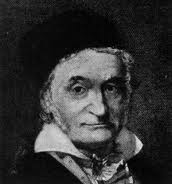(Image from scienceworld.wolfram.com).

So oftentimes, theoretical results in mathematics turn out to have completely unexpected applications. This has happened with a number of results in the field of mathematics known as number theory. Number theory studies the properties of the natural numbers: 1, 2, 3,… You might also think of them as the “counting numbers”. While they’re deceptively easy to conceive and understand, their study has gained its reputation as the “queen of mathematics”, and many of the greatest mathematicians have devoted study to their properties, which are not as simple as you might expect.

That’s weird. What could be more simple than the natural numbers 1, 2, 3,…?

You’re right! They’re easy to conceptualize, but their sometimes mind-boggling properties actually have crucial applications in the modern world, which we’ll see in this article. In the age of the internet, when we use the internet to conduct business transactions, check our bank account, use credit cards to buy things online, and take cash out of an ATM, security technologies such as armed guards, armored trucks, and X-ray machines simply don’t work. The danger with modern internet transactions is that you must send private data through a public network in order to reach its destination, such as your bank. The problem with this is that anyone can intercept read the message you have sent.

An internet-based economy therefore requires a new kind of security technology in order to protect information people send online. Actually, the field of information protection – known as “cryptography”, or “hidden writing” – isn’t new. It dates back several thousand years.

## The key ideas behind message encryption

### Our first example: the Caesar cipher

To illustrate the idea behind information protection, let’s look at a simple way to protect a message that’s being sent: an encryption mechanism known as the shift cipher. It works like this. Suppose you want to send a message to a friend, but don’t want anyone other than your friend to be able to read the message. One way to do this is to agree with your friend on a way to encode or disguise the message you will be sending, and then send the message, so that your friend, and only your friend, will know how to decode it.

The shift cipher is a simple way to encode a message: to use it, we simply shift each letter in the message by a certain, predetermined number of letters. For example, the cipher used by Roman emperor Julius Caesar to communicate with his generals was a shift cipher created by shifting each letter by three letters.

Caesar’s cipher would encode A as D, B as E, C as F, and so forth. It would encode W as Z, X as A, Y as B, and Z as C, as well. So, for example, we would encode the message, “Meet me at the restaurant” as “Phhw ph dw wkh uhvwdxudqw”, simply by taking each letter in the message and encoding it as the third letter after it.

You can probably see how to decode such a message once it is received – simply shift each letter in the message back by three letters. Got it?

I understand, but it seems so simple that it wouldn’t work. Someone could just guess how the encoding works, right?

You’re right. The problem with the shift cipher is that it is fairly easy to break. If someone captures your message before it is received by your intended recipient, they will find it easy to break, especially using a computer.

One way they could figure out how you encoded your message is by looking at the frequencies of the letters in your encoded message. The 7 most common letters, according to a study completed at Cornell University, are e, t, a, o, i, n, s, and so on – see the graph below.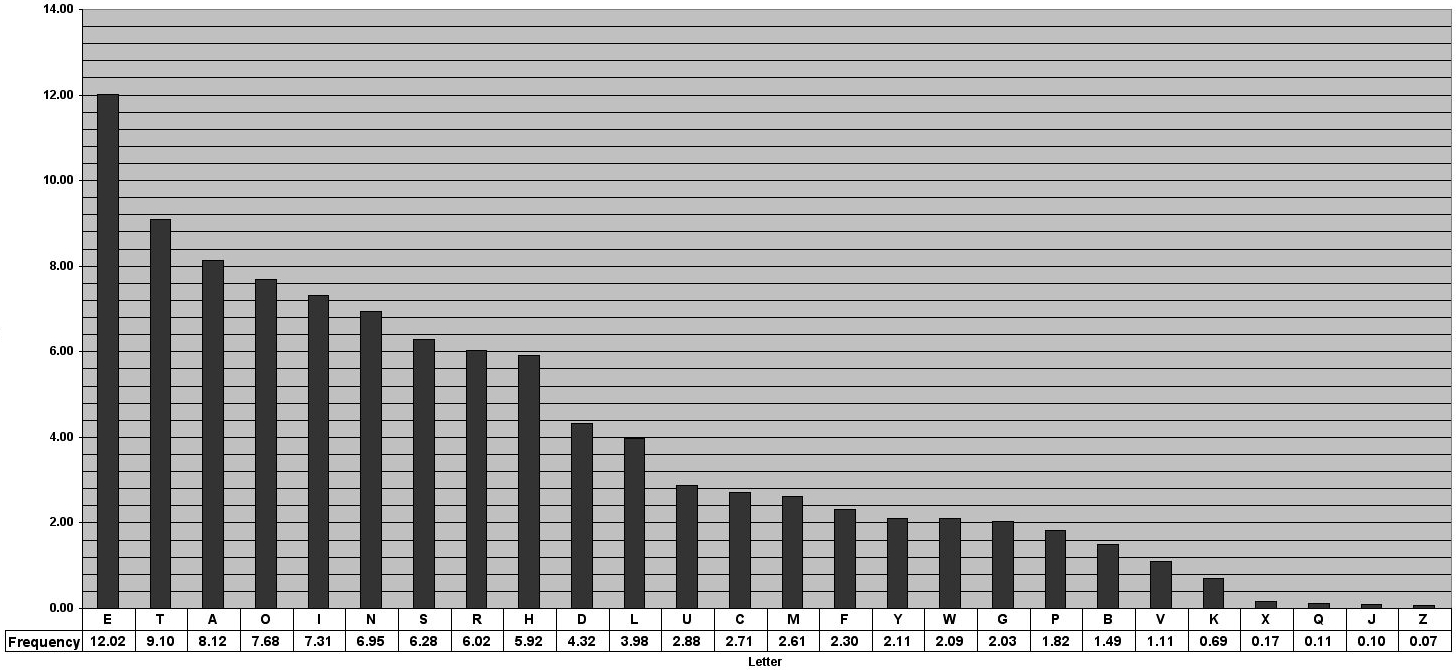By analyzing which letters appear most frequently in the encoded message, it’s pretty easy to break a shift cipher. Notice, for example, in the encoded version of our message, “Phhw ph dw wkh uhvwdxudqw”, the letters ‘h’ and ‘w’ appear frequently – because they stand for ‘e’ and ‘t’, the two most commonly used letters in the English alphabet.

Cryptographers have worked to find better methods for encoding messages – and cryptanalysts have been able to analyze or break every single method of cryptography developed in the past two millennia. A famous example of this occurred during World War II, when British mathematicians, lead by the influential computer scientist Alan Turing, were able to break the German code-making machine known as ENIGMA.

## Prime numbers and Fermat’s Little Theorem

The computer age has presented new challenges to cryptographers due to the computational power a computer possesses. The computer can quickly and easily break code-making techniques developed prior to the electronic age. This is where the work by mathematicians such as Fermat has come into play. A team of mathematicians working in the 1970’s were able to use discoveries made several hundred years before to produce a powerful method of encryption that is very difficult for even the most powerful supercomputers to break.

To see how this method, known as the RSA algorithm, works, we need to first look at some basic results of number theory, the study of the natural numbers 1, 2, 3, etc. Let’s specifically examine the subset of the natural numbers known as the prime numbers. The prime numbers are those natural numbers which have no divisors other than 1 and themselves. For example, 2, 3, and 5 are prime, while 4 and 15 are not prime, since 2 is a divisor of 4 and 3 is a divisor of 15. Here’s a table of the first 100 natural numbers. The prime numbers are in the white squares.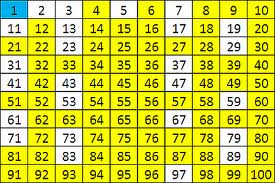(image from http://technomaths.edublogs.org/category/number/)

The prime numbers are easy to define and understand, but also turn out to have some surprisingly complex properties. The ancient Greeks were able to prove that the number of primes is infinite – that there is always another prime number. More precisely, they were able to prove that, given any finite list of prime numbers, there is always another prime number not on that list.

Mathematicians such as Fermat, Euler and Gauss, have devoted a considerable portion of their work to studying the properties of the primes, such as what’s known as the Prime Number Theorem, which tells how to estimate the number of prime numbers smaller than one million, or one billion, or any other number. More recent work has been devoted to finding large prime numbers . The largest known prime number has close to 13 million digits! It was discovered in 1008 on UCLA computers, and its finders were awarded a $100,000 prize for their work!$100,000 for finding a prime number? That seems like a ridiculous waste of money!

Well, there’s a reason that large prime numbers are considered so valuable: for one, they can be used for internet security purposes, as we shall see. Also, showing such a large number is actually prime is a feat of computer engineering that relies on networking many computers together. So, by doing this work, computer scientists are showing how the combined power of many different computers can be linked together to solve a problem.

That makes sense then. So how do large prime numbers help in ensuring computer security?

Great question. To see how prime numbers can be used to ensure internet security, let’s discuss a few basic properties about prime numbers. One of the central results in number theory pertains to the properties of prime numbers, and is known as Fermat’s Little Theorem. There’s a more famous theorem of Fermat’s, known as Fermat’s Last Theorem, which received a lot of media attention due to the amount of work required to prove it, but Fermat’s Little Theorem is probably more important in our day-to-day lives because it was a crucial step in the development of the RSA algorithm, which enables us to make secure transactions via the internet or ATMs.

So first let’s state Fermat’s Little Theorem, and then try to understand what it means. Keep in mind that for mathematicians, a theorem is simply a true statement. It’s something that mathematicians know to be true. Fermat’s Little Theorem deals with a fundamental property of prime numbers:

If p is a prime number, and a is any natural number, then the following holds: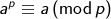Fermat’s Little Theorem states that for any prime number p and any natural number a, the above formula holds. Another way to state this is: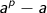is always a multiple of p, whenever p is prime and a is any natural number.

OK, so what are all those funny looking symbols?is an expression of modular arithmetic. It is not concerned with equality (which is represented by two lines, one on top of the other), but instead is concerned with the remainders left over after performing division.

The left-hand side is read as “a to the p power” – this represents the number we get by multiplying a by itself for p iterations: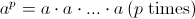Inside the parentheses on the right, the “mod” is short for “modulo”, and refers to what happens when we divide the term on the left by p. The a to the right of the three lines represents the remainder left over when we divide by p.

So this entire statement simply says that when we take a, multiply it by itself p times, and divide this number by p, we always will end up with a remainder of a; in other words,is always a multiple of p.

If you’re interested in how to prove this fact, check this page for a few different proofs of Fermat’s Little Theorem.

OK, but I’m still not convinced. Can you show an example of what you’re talking about?

Sure – let’s try this out with a few examples. Suppose we take p = 3, a prime number, and a=4. Thensimplifies toIn this case, Fermat’s Little Theorem implies that 60 is a multiple of p=3, which you can verify on your own.

Similarly, let’s take p = 11, a prime number, and a=6. Thensimplifies to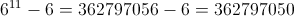.

Once again, Fermat’s Little Theorem guarantees that this number is a multiple of p = 11, and you can check this on your own.

Now, Fermat was able to prove, or demonstrate the truth of, his Little Theorem during his lifetime. At this point, however, there were no obvious real-world applications for his work.

## Fermat’s Little Theorem and the RSA Algorithm

It was only in the middle of the 20th century that Fermat’s Little Theorem became useful. At this point, relatively primitive computers were being used – they sometimes took up an entire room, and generated huge amounts of heat. Check out the picture of the early computer known as UNIVAC, below. If you get the chance to speak with scientists who were working at this time, they’ll be able to tell you stories about how time-consuming it was to run computations with these early computers. Even in these days, though, the computers were able to break primitive forms of ciphers.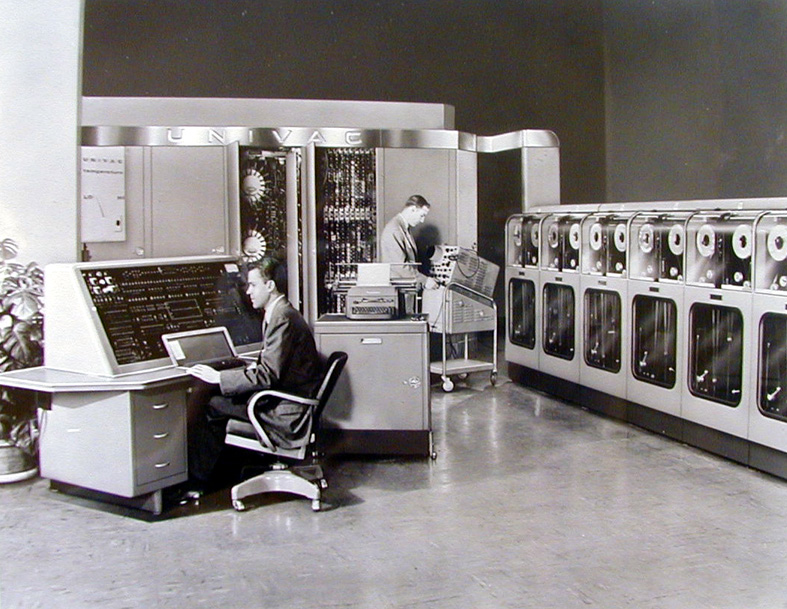image from http://www.computermuseum.li/Testpage/UNIVAC-1-FullView-B.htm

With the advent of the computer age, mathematicians began to develop new types of cryptography that would be strong enough to resist being broken by a computer. One of the most useful types of cryptography developed is known as public-key cryptography, which is used in the present day for internet security. Public-key cryptography involves both a public key – known to both the sender, the receiver, and anyone who intercepts the message in between – and a private key, known only to the sender and the receiver.

In the 1970’s, a trio of mathematicians, Rivest, Shamir, and Adelman, at Massachusetts Institute of Technology, were able to use Fermat’s Little Theorem to come up with the public-key encryption scheme known as the RSA algorithm, which is the standard encryption method used for online transactions today.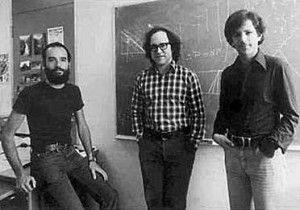MIT scientists Adi Shamir, Ronald Rivest, and Leonard Adelman, courtesy of the American Mathematical Society

The RSA algorithm consists of (1) an encryption procedure, which is publicly known, and (2) a decryption procedure, which is only known to the sender and the receiver.

Here’s how it works. When you send information via electronic means, such as at the ATM, the computer converts your information – the message you want to send – into a single number. You might try to think of a way to do this on your one, but to start you might simply designate a as 01, b as 02, and so forth. Call the number representing your message m.

Now – here’s the key idea behind the RSA scheme – we want to find numbers e and d (for encryption and decryption) such that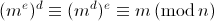(recall this means that if we subtract m from either of the quantities on the left, what’s left is a multiple of n). If we can find such numbers, then raising our message, m, to the e power – that is, multiplying m by itself e times (and finding the remainder modulo n) – will correspond to encoding our message, and raising the encoded message to the d power (modulo n), corresponds to decoding the message.

To carry out the RSA encryption, we then use two large prime numbers; prime numbers of around 100 digits should be sufficient. Call these primes p and q. Form the product of the two primes, and call this number n, so that n = p * q. Also form the number z = (p-1)*(q-1). (The number z is important because it represents how many numbers between 1 and n do not share factors with n).

To find such numbers, we can recall Fermat’s Little Theorem, which suggests that, for any prime number p,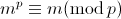If we pick e to be any prime number less than z that is not a divisor of z, then we are able to find a number d such that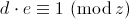We can find such a number using a process known as Euclid’s algorithm. A bit of arithmetic, using Fermat’s Little Theorem, will show that once we have found d and e in this manner, thenSo, we encode the message using the encryption number e, send the encoded message, together with e and n, over the public domain, while keeping d known to only the sender and the receiver. This ensures the security of the message since a hacker would calculate the factors of n, the product of two large prime numbers, in order to find d, which would take a modern computer many decades to complete. The prime numbers p and q used to calculate n are changed sufficiently often to ensure that the RSA algorithm is secure.

Here’s a diagram representing the process used in RSA for encrypting and decrypting our message: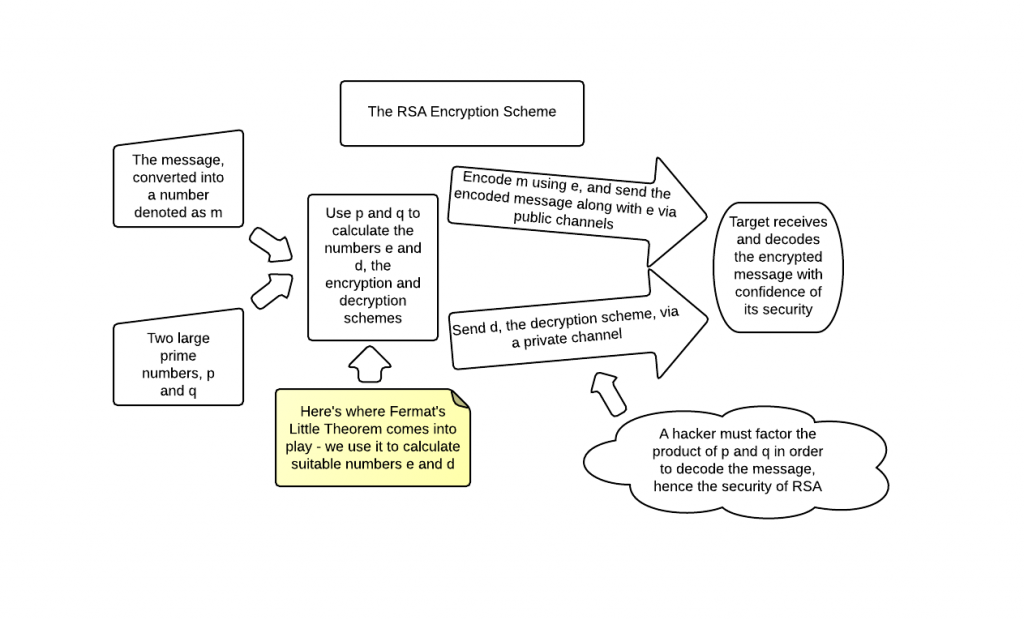To illustrate this, let’s carry RSA out for a real “message”. Suppose we want to “send” the number 23 in an encrypted fashion.

First, we pick two large prime numbers. A real-life RSA encryption scheme might use prime numbers with 100 digits, but let’s keep it simple and use relatively small prime numbers. Take p=47 and q=43.

Now we form the product n=p*q=47*43=2021, and the number z=(p-1)*(q-1)=46*42=1932.

At this point we’re ready to find our actual encoding and decoding schemes. We want to find numbers e and d such that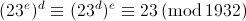.

First, let’s try to find a prime number e that is not a divisor of 1932. Let’s take e=17.

Now we compute d using the Euclidean algorithm. We use this algorithm to find a number d such that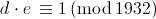We find that d=341 will work. You can check on your own that 341*17=5797, while 1932*3 = 5796, so 341*17 leaves a remainder of 1 (modulo 1932).

So, to encode our message m=23, we simply send the “message” attained by encrypting 23 using e: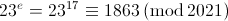So our encrypted message is 1863, and we also also send e=17 and the number n=2021 with the encrypted message, while maintaining the privacy of d=341 to ourselves and the receiver. Now we come to the key point. If a hacker wants to decrypt the message, s/he must calculate d, which in this case would entail factoring 2021 to p and q. This is easy for a computer to complete in this example, but very time consuming for large choices of p and q.

The receiver, however, knows d, which allows him/her to perform the following operation to recover the initial message: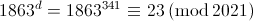.

## To sum it all up…

Now, we’ve covered some fairly heavy-duty mathematics.  The basic idea behind RSA algorithm is: first, we convert a message we want to send (such as bank account or credit card information) to a number, designated as m.  We then pick two large prime numbers, p and q, and from these numbers we calculate (based on Fermat’s Little Theorem) two numbers e and d, respectively the encryption and decryption schemes.  We use e to encode m, then send this encoded message over the public domain.  We keep d private, known only to ourselves and our intended destination.  Now, someone who intercepts the encoded message and tries to decode it will have to factor a very large number formed by the product of p and q.  For large values of p and q, such as prime numbers with 100 digits, this might take a modern computer 100 years.  Our message is therefore, in practice, secure.

If you’ve made it through all this – congratulations. This is university-level material. If you’re interested in reading more of the heavy-duty mathematics behind the RSA algorithm, check out this paper the mathematician Yevgeny Milanov.

I’ve chosen this as a first article to write here on science4all.org because it serves as a good example of how a topic in pure mathematics can turn out to have a completely unexpected application – the type of unexpected connection that often results in scientific discoveries and is known as serendipity.  In this case, discoveries about prime numbers culminating in Fermat’s Little Theorem gives us a way to encrypt a message and send it through a network securely, so that only its intended target, who has a decryption mechanism, can read the message.

Recall that the main reason the RSA algorithm is so strong is the fact that, in order to break it, it’s necessary to find the prime factors of a gigantic number, a number with around 200 digits. This might take a supercomputer 100 years to complete!

However, computer scientists are busy working on a quantum computer, a computer that would use the states of an electron as its computing components, rather than the ‘0’ and ‘1’ bits current computers use.

If they are successful in implementing a quantum computer, it will be very easy to find the prime factors used in the RSA algorithm. So a much stronger algorithm will be necessary to maintain network security in an age with quantum computers.

Amazingly, mathematicians working in the topic area known a “knot theory”, which makes a formal study of the knots we use to tie shoelaces or ropes, have developed an algorithm that uses an analogy of prime numbers, called “prime knots”, to develop a public-key cryptography method that is immune to quantum eavesdroppers. Amazing!

## More on Science4All

Cryptography and Quantum PhysicsBy Scott McKinney | Updated:2016-02 | Views: 2787
Recent discoveries in the branch of physics known as quantum mechanics have powerful applications in the field of network security - they have the potential to break forms of internet security based on mathematics such as the RSA algorithm, and also present new ways to safely send information. In this article we’ll see how a physics-based method can be used to secure online information.

P versus NP: A Crucial Open ProblemBy Lê Nguyên Hoang | Updated:2016-01 | Views: 7259
P=NP is probably today's most crucial open problem. Not only is it a very theoretical question in computer science and mathematics, but it also has major implications to real world. Its resolution could revolutionize the world. This article gives the definition and major results of P=NP.